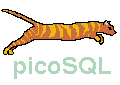## picoSQL - Language reference manual## 8) Query optimizer

picoSQL use a query optimizer which find the best way to satisfy the required queries. Only two read startegies are implemented, the sequential scanning of the whole table and the use of an appropriate index.

picoSQL find the best startegy analyzing the search conditions, so it is very important to define appropriate indexes and to use search condition that can use them, especially on large tables.

To show, in a simple way, how picoSQL works, we suppose now to have a table with only one index of a single column, whose name is I.

The query optimizer analizes the search condition only in a formal way, that is without taking in consideration the effective values neither the values really stored in the table. To understand why it acts in this way, it must consider that often the queries contain dynamic parameter, as in the following example:

I = ?

This query can be executed more than one times, substituting a different value in place of the question mark. Clearly in such case, it is more efficient to analize the query only one time and to find a searching strategy that is good for any parameter value.

To repeat the analisys each time the value change is more expensive and usually do not improve the strategy.

On this base, picoSQL is able to find a good search index, if one exists.

To use an index for searching some data, the search condition must characterize one or more exhaustive intervals on the columns of the index, containing all the required rows, as in the following example:

I < 5

Because the query optimizer do not consider the data on the table, sometimes the use of an index do not improve the performances: for example, if the column I contains only values that are less than 5, the previous search condition do not limit the number of required rows, so a sequential scanning would be more efficient.

To put any condition in AND to a condition that characterize an exhaustive interval, do not influence the goodness of an index, so, from the optimizer point of view, the following condition is as good as the previous one.

I < 5 AND A > 10

Things are different changing the AND operator with the OR operator. In the following condition:

I < 5 OR A > 10

the condition on the index do not characterize all the rows required, so the interval is not exhaustive and the use of the index is not useful. To have an index defined on the A columns do not change the situation because not even A characterize an exhaustive interval. To read both the indexes, eliminating the duplicate rows, is expensive and can cause to scanning the whole table two times in the worst case. So, in such situations, the optimizer prefers to scan the whole table one time.

From this observation, we can derive a simple rule of thumb: if you use the OR operator, probably the whole table must be scanned. However, this is not true for any query: in some cases the optimizer is able to find exhaustive intervals also if there are OR operator in the search condition, as in the following example:

I < 5 OR I > 10

In this case there are two intervals, but they contain all the required rows. picoSQL is able to take advantage of indexes when there are more than one exhaustive interval, also if they are overlapped, as in the following case:

I > 5 OR I > 10

When an index is composed by more than one column, the theory is the same but to understand if the optimizer can take advantage of the use of an index is more difficult.

The optimzer can take advantage of the nth part of a composed index if n = 1 or if the optimizer can take advantage of the (n - 1)th part. We suppose now to have a composed index on the columns named I, J and K. Lets look the following search conditions.

I > 5 AND J < 10 AND K = 4

I > 5 AND K = 4

K = 4

In the first search condition the optimizer can take advantage of all parts of the index, in the second one the optimizer can take advantage of only the first part of the index while in the third one the optimizer cannot use the index at all.

Now we can combine this new rule with the previous one to understand if an index can be used or not. Lets look the following search conditions.

(I = 5 AND J = 10 AND K = 4) OR (I = 6 AND J = 11 AND K = 5)

(I = 5 AND J = 10 AND K > 4) OR (I = 5 AND J > 10) OR I > 5

In the first one the optimizer can take advantage of all three part of the index while in the second one the optimizer can take advantage of only the part on column I; in fact in this case J and K can have any values, so they cannot used as indexes. In thruth, if we analyze the values, we can characterize an exhaustive interval, but, to do this, we need to considers the cotextual values and picoSQL do not do this.

Index Previous